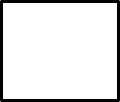1
visibility

Determine the number that makes the equation true.

21 + 18 = 50 –• A

14

• B

15

• C

19

• D

11

The correct answer is 11.

Solution:
Consider the given equation.
21 + 18 = 50 –To determine the missing number, we need to find: 21 + 18.

Which gives:
21 + 18 = 39

So,

21 + 18 = 50 –39 = 50 -Next, we look for the number which when subtracted from 50 gives 39.

Note that,
50 - 11 = 39

Then,
21 + 18 = 50 - 11

So, number 11 makes the equation true.